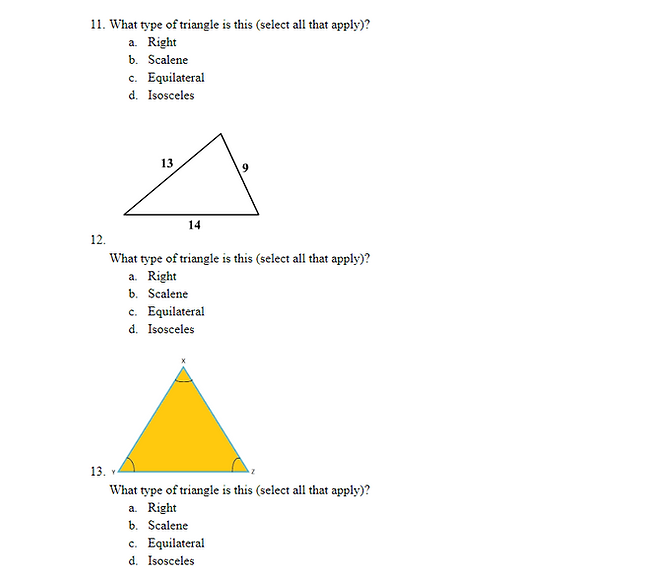top of pageGeometry

Welcome to the guide types of angles and triangles

This is an introductory lesson on Triangles. If you feel that you have  a good understanding of triangles, test your knowledge to confirm you know 100% of the material. If not, do not worry and follow the lesson closely!

Background Concepts:

Types Of Angles:

https://www.mathsisfun.com/angles.html (This is helpful because you can play around with the angles)

Triangles:

https://www.mathsisfun.com/triangle.html (Notice what happens to the other two angles when you change one angle of the triangle)

https://www.khanacademy.org/math/geometry/hs-geo-trig/hs-geo-special-right-triangles/v/45-45-90-triangles (Special right triangles PART 1)

https://www.khanacademy.org/math/geometry/hs-geo-trig/hs-geo-special-right-triangles/v/intro-to-30-60-90-triangles (Special right triangles PART 2)

WAIT: Can there be more than one obtuse angle in a triangle? What about right angles? Acute angles?

The angles of a triangle add up to 180 degrees (remember the formula 180 * (n-2)).

Since obtuse angles are greater than 90 degrees, two of these angles would add up to be greater than 180. Since 2 right angles add up to 180 degrees, it doesn’t leave room for a third angle. Therefore, you cannot have two obtuse or two right angles in a triangle. However, you can have two acute angles because they add up to less than 180 degrees.

Practice:

https://www.ixl.com/math/geometry/special-right-triangles (Slightly more challenging, you can stop if you get to a problem that requires trigonometry (which would be giving you three angles but no side lengths)

_____________________________________________________________________________________________________

Check for Understanding (final review):